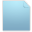###objects mass and forces units

05 Apr 2016 15:15 #1

1. What are the units of the ODE objects mass? for examples in the demo it is 0.04.

2. I'm trying to apply field force on the tool using:

and then on the cube using:

in the two cases, the force on the haptic device (when I lift the cube with the gripper) is different.

my question is, are the units the same in both cases? because the forces are different with a constant scaling factor. (in the second case the force is larger)

08 Apr 2016 00:34 #2

The different demonstrations use MKS system of units. However the forces that are applied to the hapttic device are sometimes scaled in order to adjust for force limitations of the haptic devices as well as different workspace scale factors that need to be applied.

In example 01-ODE-cube, if you wanted to normalize everything, you would modify

`ODEobject->addExternalForceAtPoint(-0.3 * interactionPoint->getLastComputedForce(), collisionEvent->m_globalPos);`

with the following:
`ODEobject->addExternalForceAtPoint(-1.0 * interactionPoint->getLastComputedForce(), collisionEvent->m_globalPos);`

Regarding the workspace, you could set the scale factor to 1.o by modifying line:
`tool->setWorkspaceRadius(1.3);`

with the following:
`tool->setWorkspaceScaleFactor(1.0);`

Depending of the haptic device that you are using, you may also need to adjust for the stiffness of each object as well as their size.

18 Apr 2016 12:48 #3

I will try to explain better my situation:
In my experiment I lift a cube that connected to a vertical spring. I want to apply force on the tool that is proportional to the length of the spring.
When I apply the force directly on the tool (using m_tool->m_lastComputedGlobalForce.add(forceField) ) the force is equal to what I want.
But I want to apply the force on the cube and not on the tool (I tried using ODEobject->addExternalForce(forceField) ) and when I lift the cube with the tool, the force I feel is something like 3 times higher, but I don't find constant relations between them.
how can I apply the force I want on the cube?

19 Apr 2016 09:58 #4

To better understand the problem, what makes you think that the forces you are applying to the object behave as if they were three times higher? Are you observing some instability issues?

19 Apr 2016 10:30 #5

I can feel that the forces are much higher, and I measured the forces using:
m_tool->m_lastComputedGlobalForce
and compared them in both cases.